# Acta Universitatis Danubius. Œconomica, Vol 11, No 1 (2015)

A Study of Allen Production

Function with Differential Geometry

Alin Cristian Ioan1

Abstract. In this paper we shall made an analysis of Allen production function from the differential point of view. We shall obtain some interesting results about the nature of the points of the surface and the total curvature.

Keywords: production functions; metric; curvature; Allen

JEL Classification: E23

1. Introduction

In the theory of production functions, all computations and phenomenons are studied for a constant level of production. In order to detect many aspects of them, a complete analysis can be made only at the entire surface.

We define on R2the production space for two resources: K – capital and L - labor as SP=(K,L)K,L0 where xSP, x=(K,L) is the set of resources. Because not any amount of resources are possible, we restrict the production area to a subset DpSP called domain of production.

It is called an Allen production function an application:

Q:DpR+, (K,L)Q(K,L)=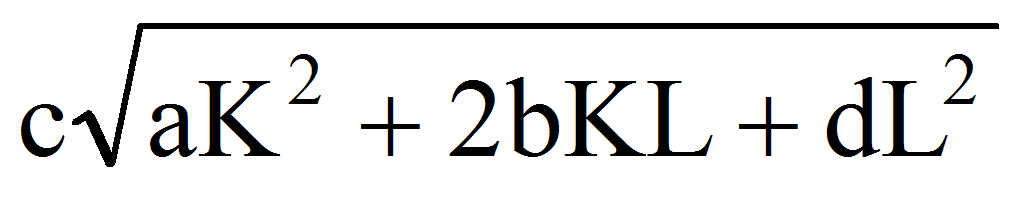R+ (K,L)Dp, a,b,c,dR*+, c0

The production function is C-differentiable and homogenous of degree 1.

2. The Differential Geometry of Allen Surface

The graph representation of a production function is a surface.

Let note in what follows:

(1) p=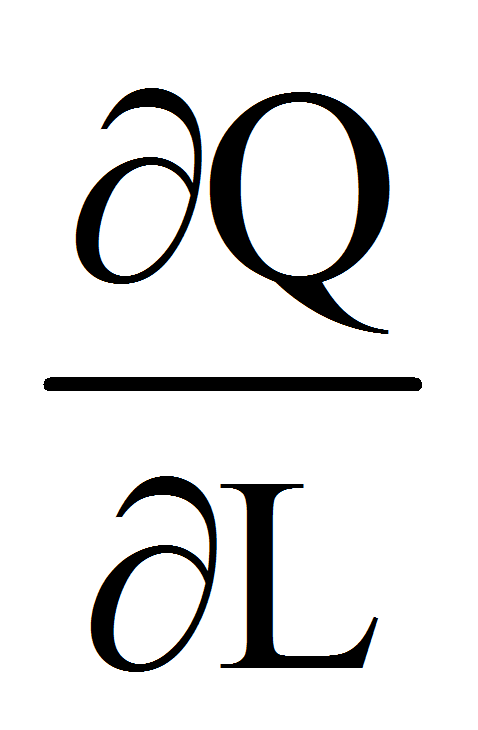, q=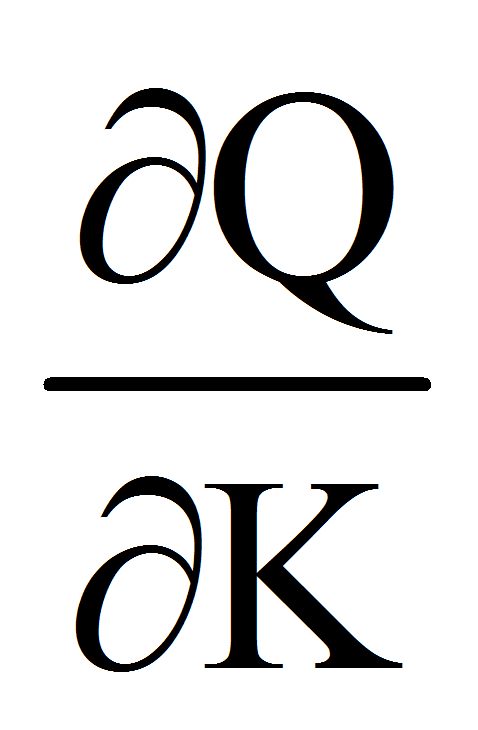, r=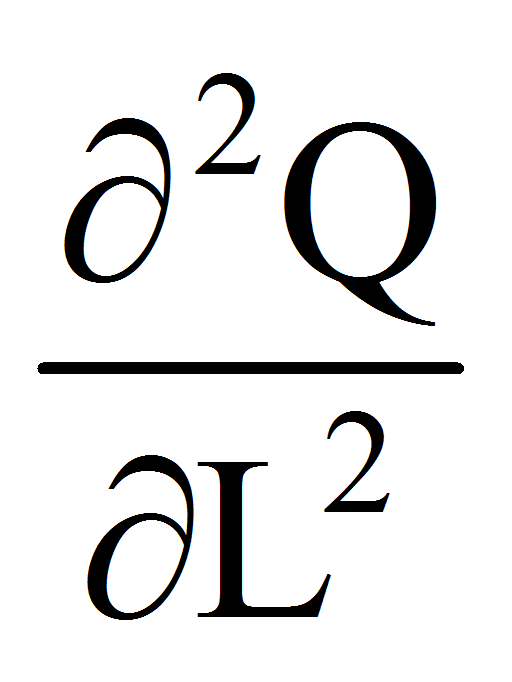, s=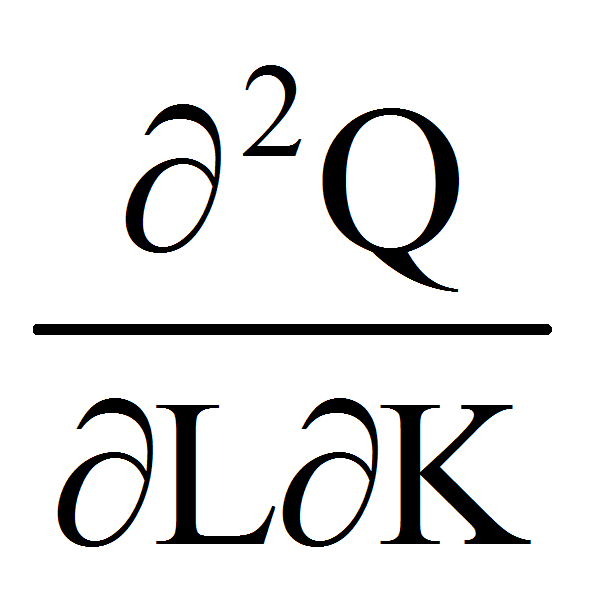, t=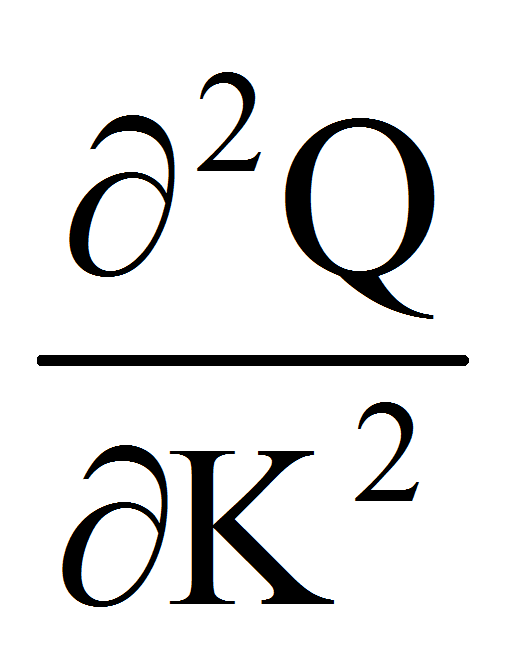We have after simple calculations:

(2) p=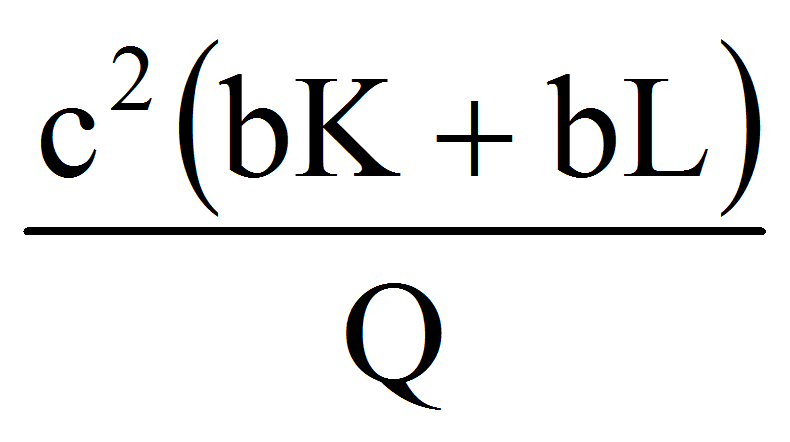, q=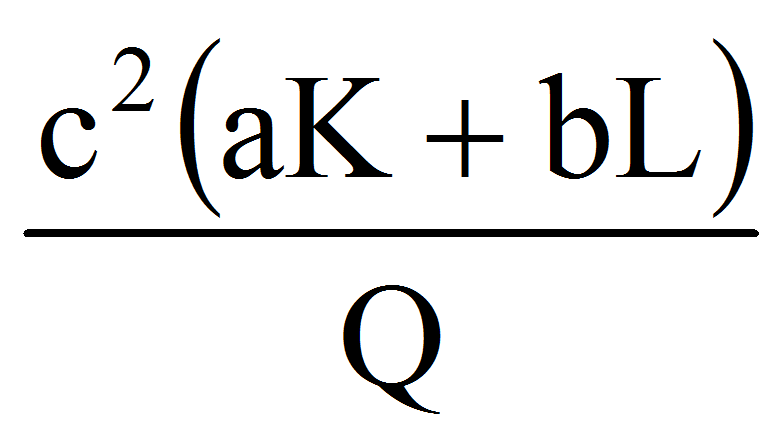, r=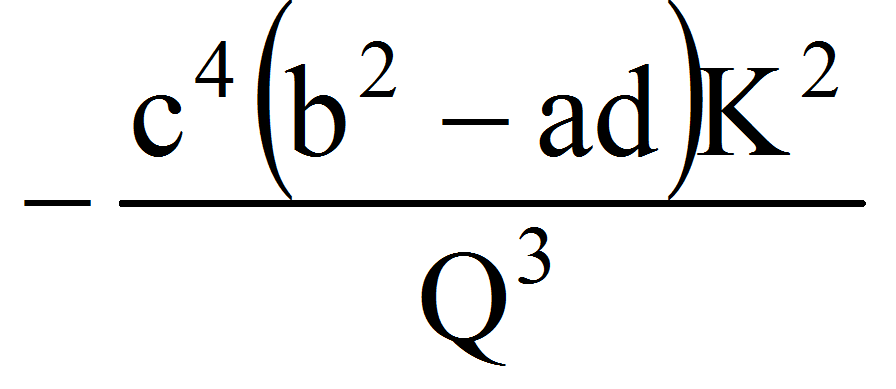, s=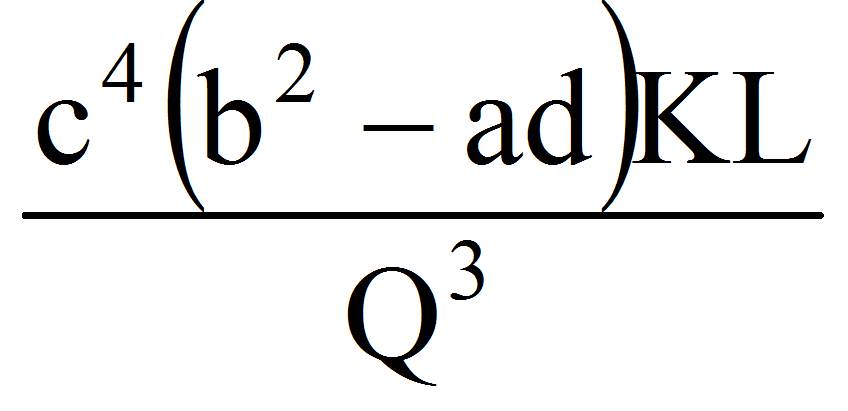, t=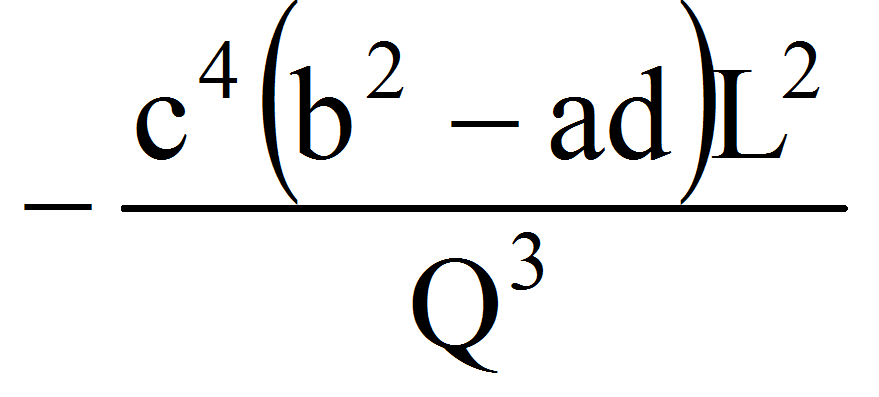The bordered Hessian:

(3) Hf=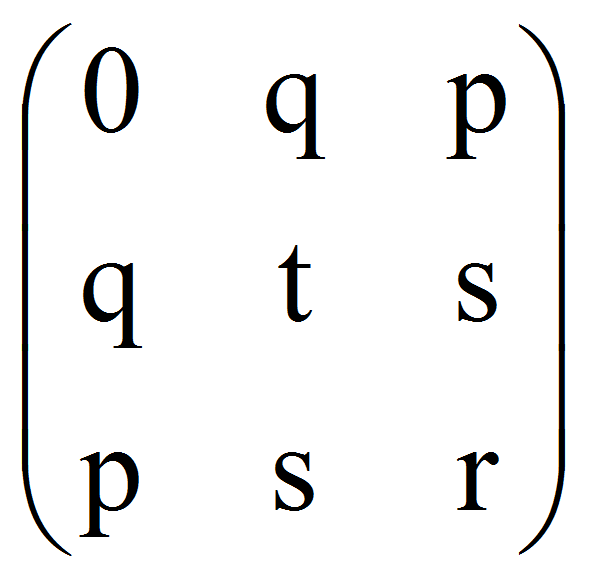=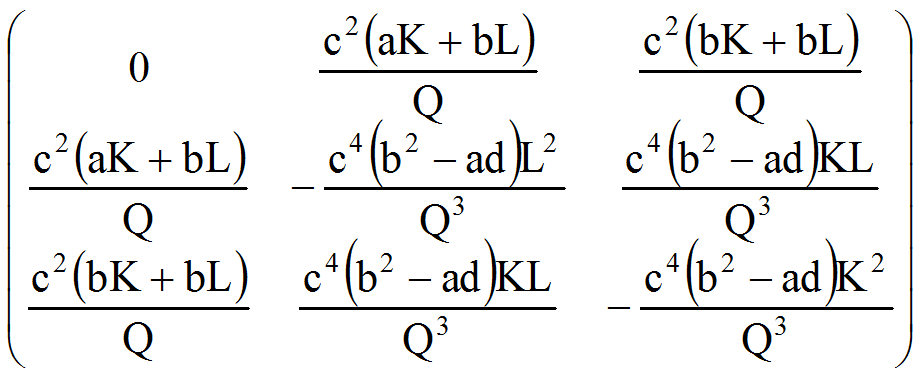therefore, because:

(4) B1=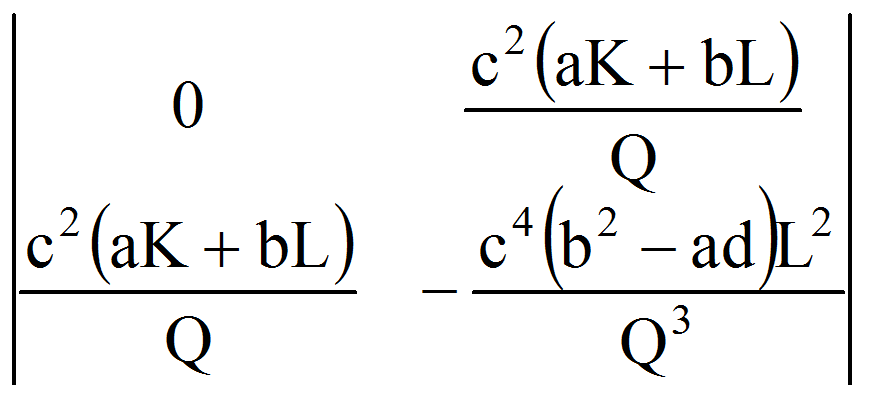=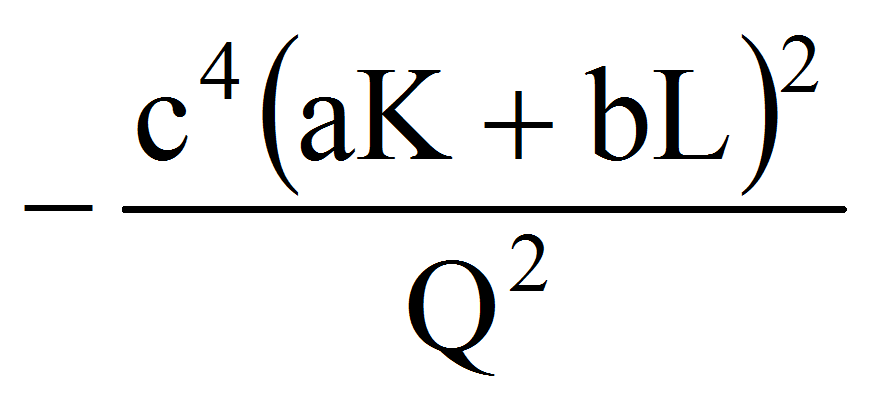0,

B2=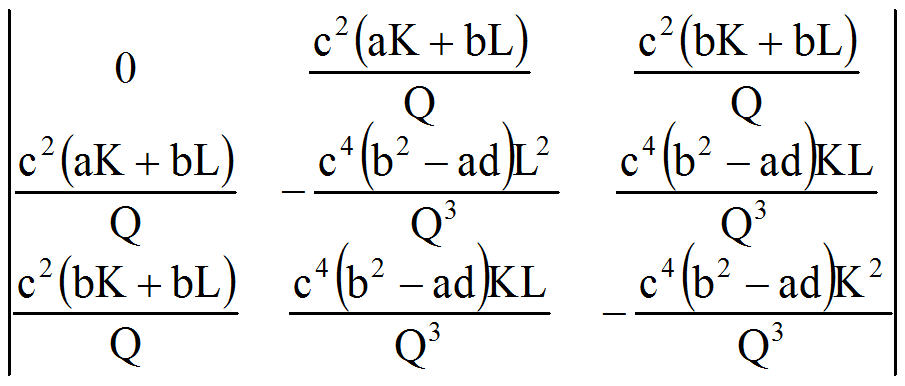=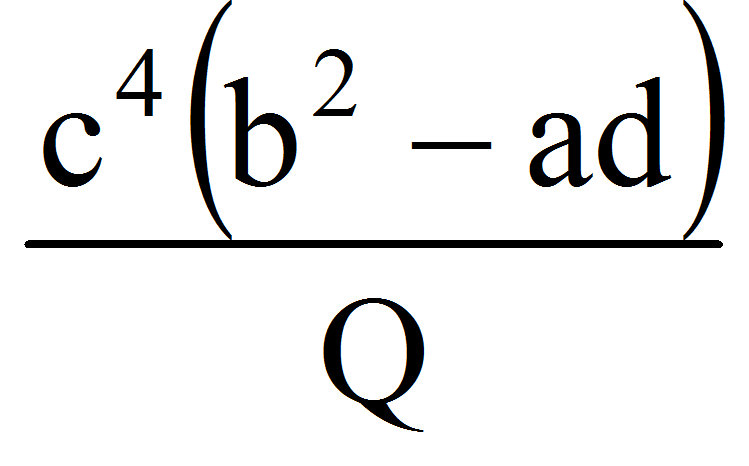we obtain that in order to Q be quasiconcave (that is for any aR, Q-1([a,)) is convex) we must have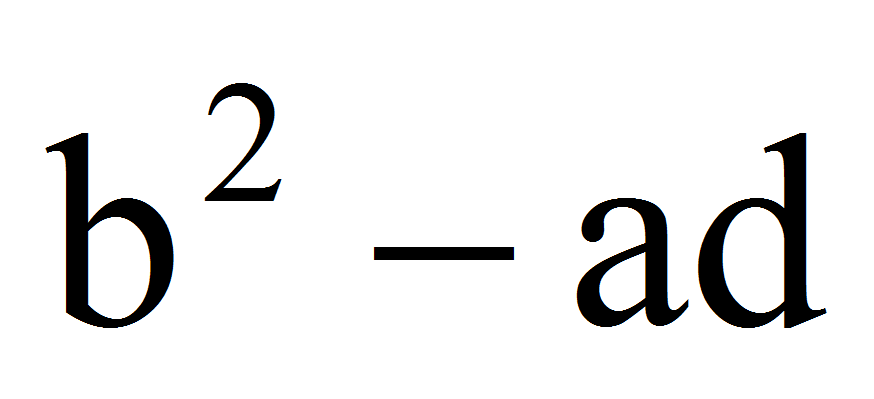0.

For a constant value of one parameter we obtain a curve on the surface, that is Q=Q(K,L0) or Q=Q(K0,L) are both curves on the production surface. They are obtained from the intersection of the plane L=L0 or K=K0 with the surface Q=Q(K,L).

In the study of the surfaces, two quadratic forms are very useful.

The first fundamental quadratic form of the surface is:

(5) g=g11dL2+2g12dLdK+g22dK2

where: g11=1+p2, g12=pq, g22=1+q2.

In our case:

(6) g11=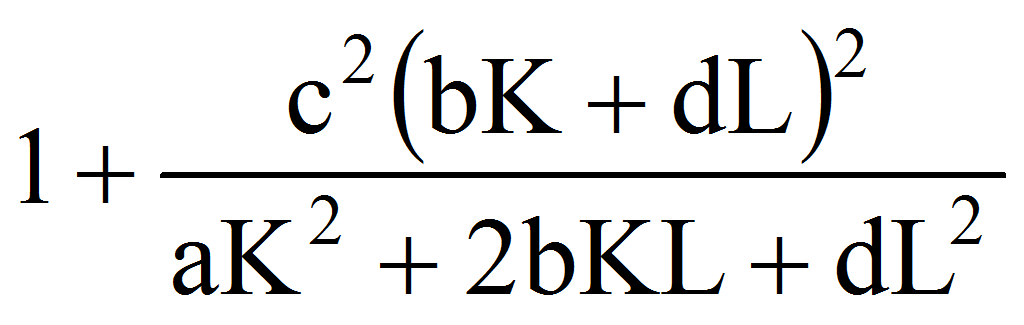, g12=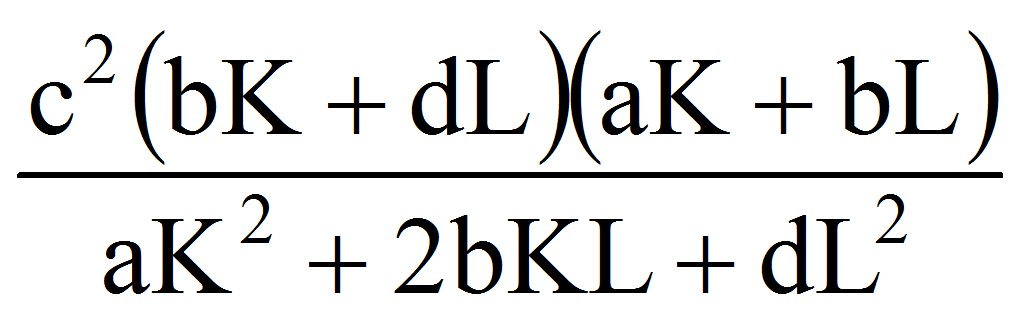, g22=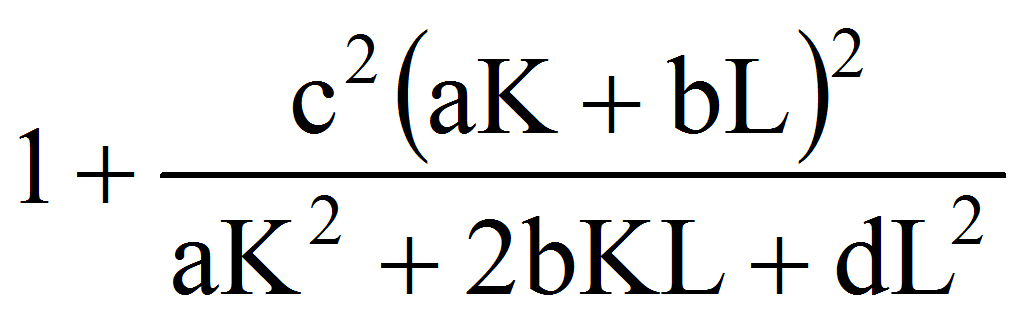The area element is:

(7) d=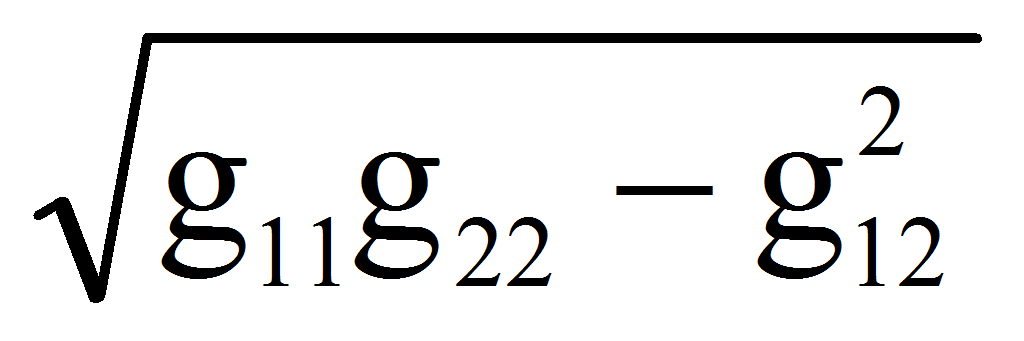dKdL=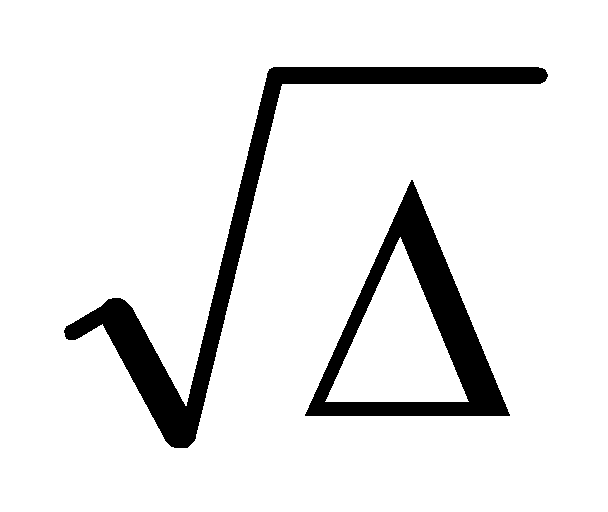dKdL=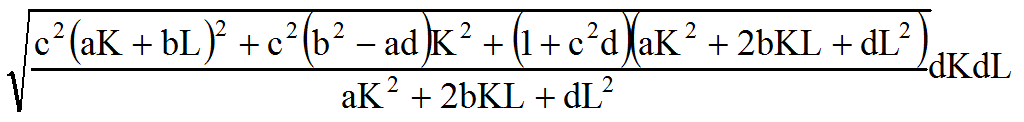and the surface area A when (K,L)R (a region in the plane K-O-L) is A=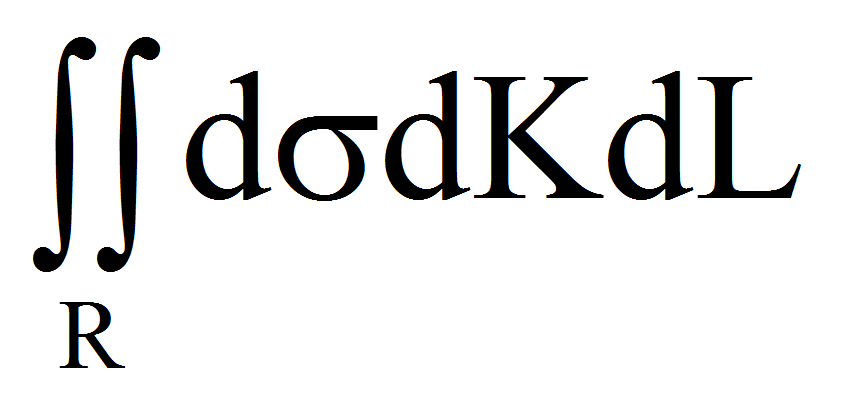.

The second fundamental form of the surface is:

(8) h=h11dL2+2 h12dLdK+ h22dK2

where: h11=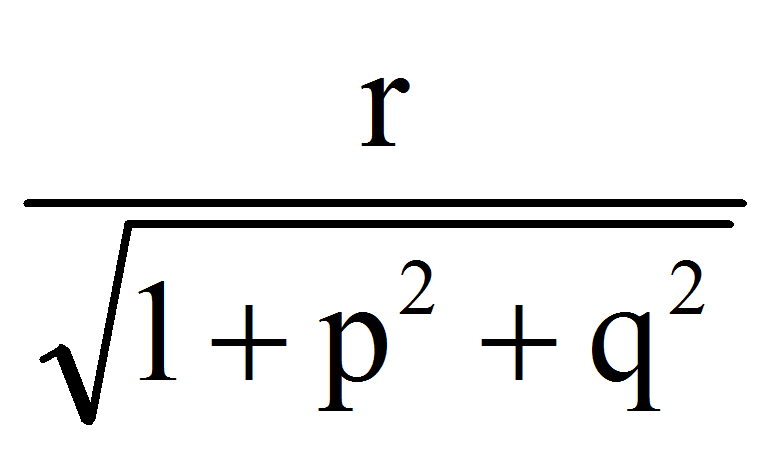, h12=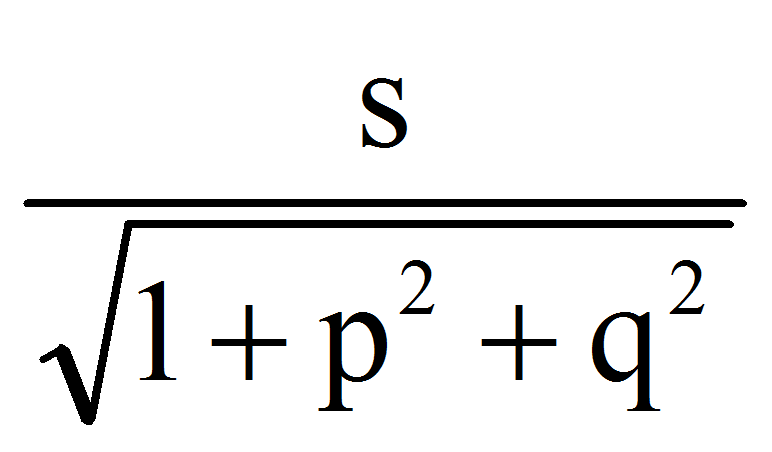, h22=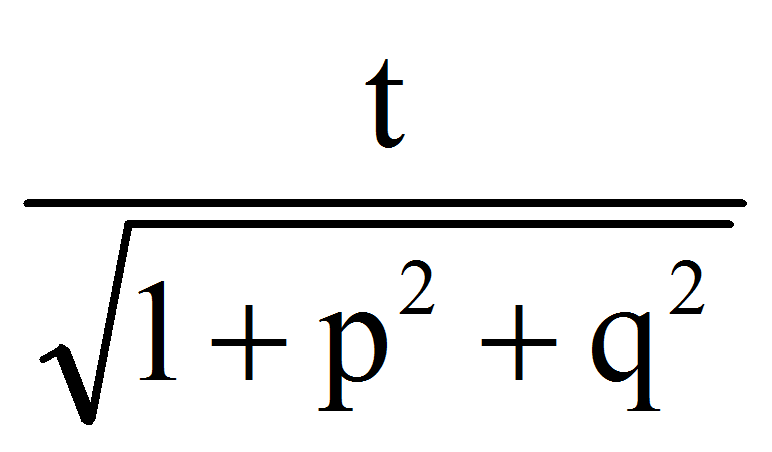.

In our case:

(9) h11=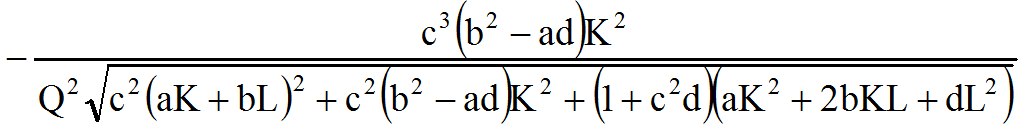,

h12=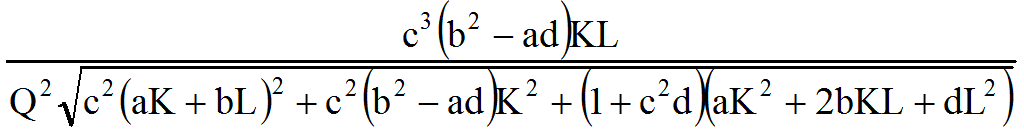,

h22=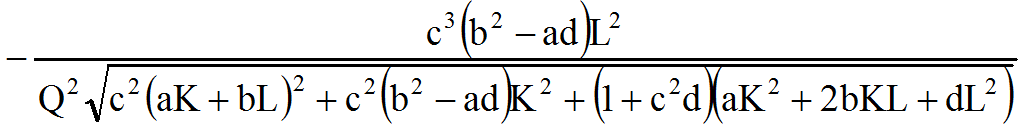.

Considering the quantity =h11h22-h122 we have that:

(10) =0

If >0 in each point of the surface, we will say that it is elliptical. Such surfaces are the hyperboloid with two sheets, the elliptical paraboloid and the ellipsoid. If 0 in each point of the surface, we will say that it is hyperbolic. Such surfaces are the hyperboloid with one sheet and the hyperbolic paraboloid. If =0 in each point of the surface, we will say that it is parabolic. Such surfaces are the cone surfaces and the cylinder surfaces.

From (10) we find that the production surface is parabolic.

The curvature of a curve is, from an elementary point of view, the degree of deviation of the curve relative to a straight line. Considering a surface S and an arbitrary curve through a point P of the surface who has the tangent vector v in P, let the plane determined by the vector v and the normal N in P at S. The intersection of with S is a curve Cn named normal section of S. Its curvature is called normal curvature.

If we have a direction m=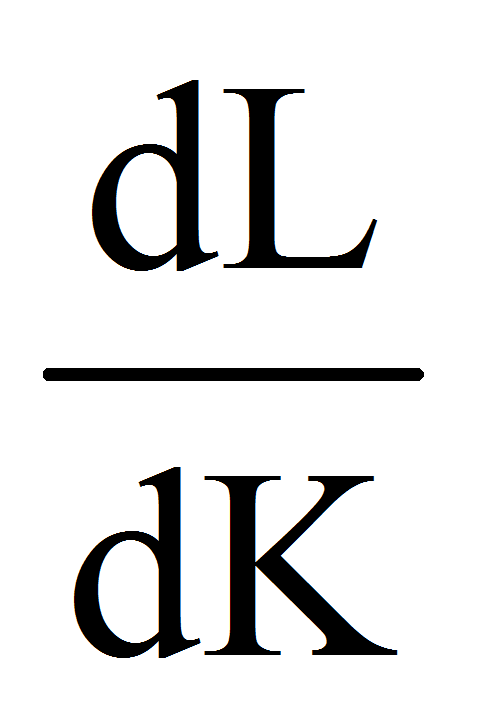in the tangent plane of the surface in an arbitrary point P we have that the normal curvature is given by: k(m)=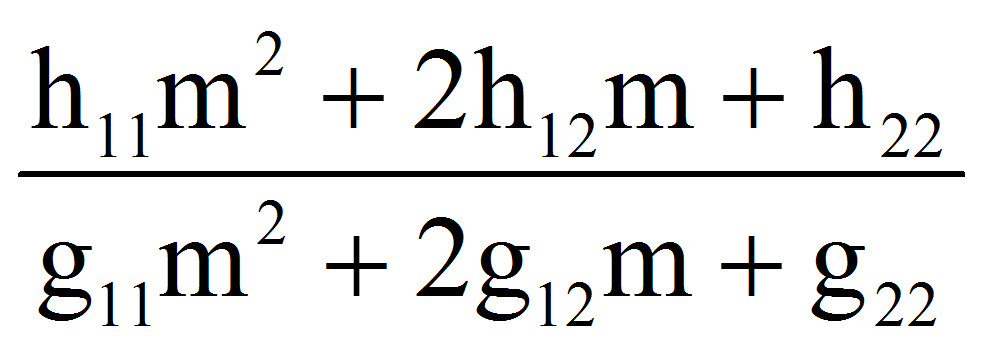. The extreme values k1 and k2 of the function k(m) are the principal curvatures of the surface in that point. The quantity K=k1k2 is named the total curvature in the considered point. We have K=0 therefore the surface has null constant total curvature.

3. References

Arrow, K.J.; Chenery, H.B.; Minhas, B.S. & Solow, R.M. (1961). Capital Labour Substitution and Economic Efficiency. Review of Econ and Statistics, 63, pp. 225-250.

Cobb, C.W. & Douglas, P.H. (1928). A Theory of Production. American Economic Review, 18, pp. 139–165.

Ioan, C.A. (2007). Applications of the space differential geometry at the study of production functions. Euroeconomica, 18, pp.30-38.

Ioan C.A. (2004). Applications of geometry at the study of production functions. The Annals of Danubius University, Fascicle I, Economics, pp.27-39.

Kadiyala, K.R. (1972) Production Functions and Elasticity of Substitution. Southern Economic Journal, 38(3), pp. 281-284.

Kmenta, J. (1967). On Estimation of the CES Production Function. International Economic Review, 8(2), pp. 180-189.

Liu, T.C. & Hildebrand, G.H. (1965). Manufacturing Production Functions in the United States. Cornell Univ. Press, Ithaca.

Mishra, S.K. (2007). A Brief History of Production Functions. North-Eastern Hill University, Shillong, India.

Revankar, N.S. (1971). A Class of Variable Elasticity of Substitution Production Functions. Econometrica, 39(1), pp. 61-71.

Sato, R. (1974). On the Class of Separable Non-Homothetic CES Functions. Economic Studies Quarterly, 15, pp. 42-55.

1 Nicolae Oncescu College, Braila, Address: 1-3 ŞOS. Brăilei, City: Ianca, Brăila County, Tel.: +40239-668 494, Corresponding author: alincristianioan@yahoo.com.

AUDŒ, Vol. 11, no. 1, pp. 115-119

### Refbacks

• There are currently no refbacks.